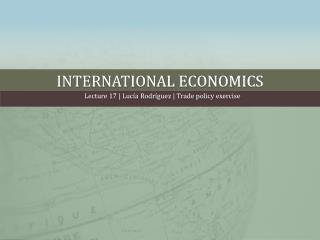DownloadDownload PresentationINTERNATIONAL ECONOMICS

# INTERNATIONAL ECONOMICS

Télécharger la présentation## INTERNATIONAL ECONOMICS

- - - - - - - - - - - - - - - - - - - - - - - - - - - E N D - - - - - - - - - - - - - - - - - - - - - - - - - - -
##### Presentation Transcript

1. INTERNATIONAL ECONOMICS Lecture 17 | Lucía Rodríguez | Trade policy exercise

2. Trade Policy • In autarky: Qdi= 140 – 20pi et Qsi = 20pi – 20 Pi*= 4  Qi*= 60 Pi 7 4 1 Good i 60 140

3. Trade Policy • The country enters free-trade: Piworld= 2  Qid= 100 butQis= 20  Imports = 80 Consumer surplus has increased by (a + b + c) = [½ * (Qi* )* (Pmax - Pi*)] – [½ * * (Qid) * (Pmax - Piworld )] = 160 Producer surplus has decreased by (a) = [(Pi*- Piworld )* (Qi* )] – [½*(Pi*- Piworld )* *(Qi* - Qis) = 80 Pi 7 4 a b c Pw=2 1 Good i 20 60 100 140

4. Trade Policy • The government sets an ad-valorem tariff of 50%: Pinew= 3  Qidnew= 80 and Qisnew= 40  Imports = 40 Prohibitivetariff: 2*(1+t) = 7; t = 250% Consumer surplus has decreasedby 90 Producer surplus has increasedby 30 Pi Pw *(1*0,5)=3 a b c Pw=2 Good i 40 60 80 140

5. Trade Policy • The government sets a quota on imports equal to 40 units • Now: Qdi= 140 – 20pi et Qsi = 20pi – 20 + 40 Pi*= 3 Pinew= 3  Qidnew= 80 and Qisnew= 40  Imports = 40 Pi • Exactly the same effect as the 50% tariff! • What would you do then, if you were asked to find the tariff-equivalent to a 40 units quota? Pw=2 Good i 20 100 140 80 40